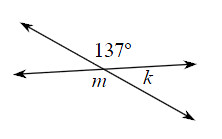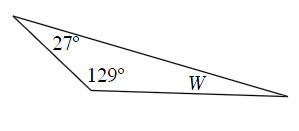### Home > MC1 > Chapter 10 > Lesson 10.2.1 > Problem10-72

10-72.

Find the measures of the lettered angles in the figures below.

1.Since $m$ and $137°$ are vertical angles, $m = 137°$.

$m + k = 180°$

$180° − 137° = 43°$
$k = 43°$

$k = 43°$

1.The angles within a triangle add to $180°$.